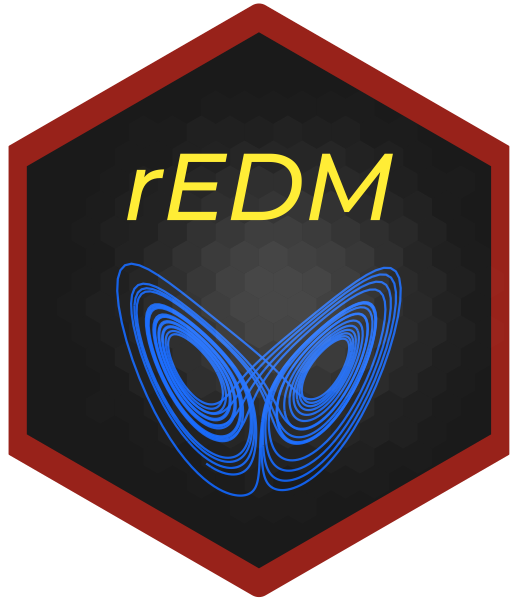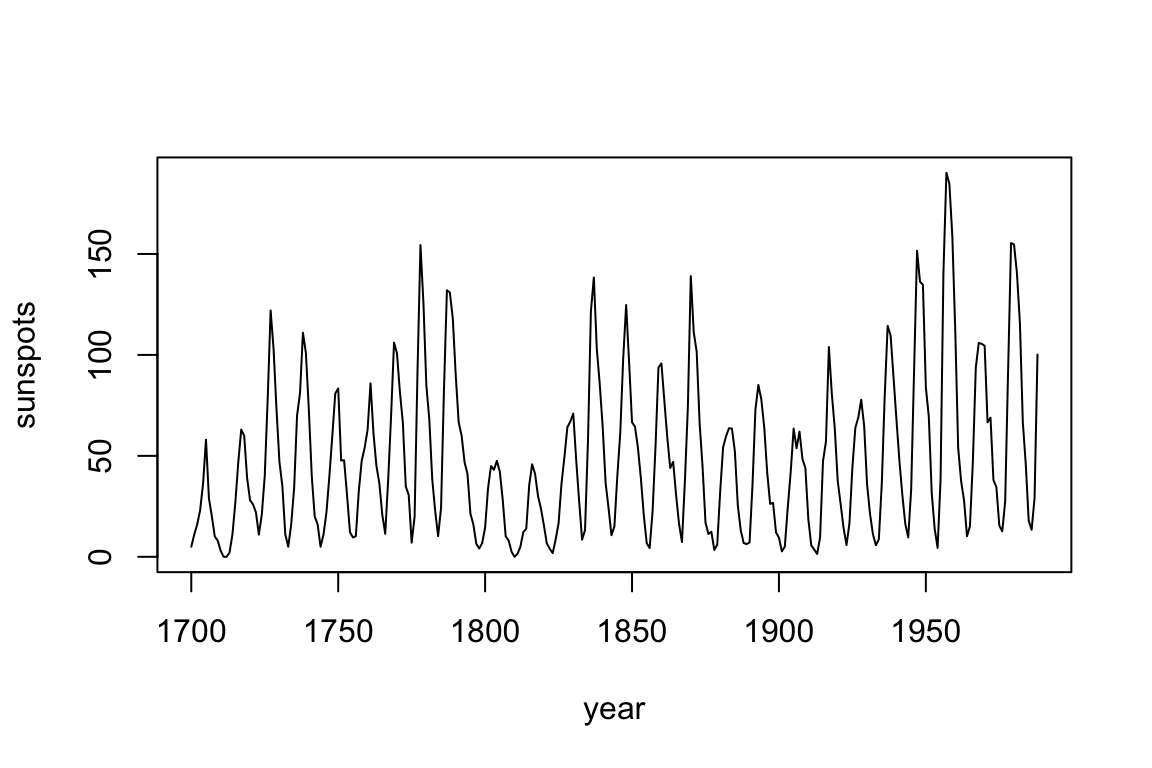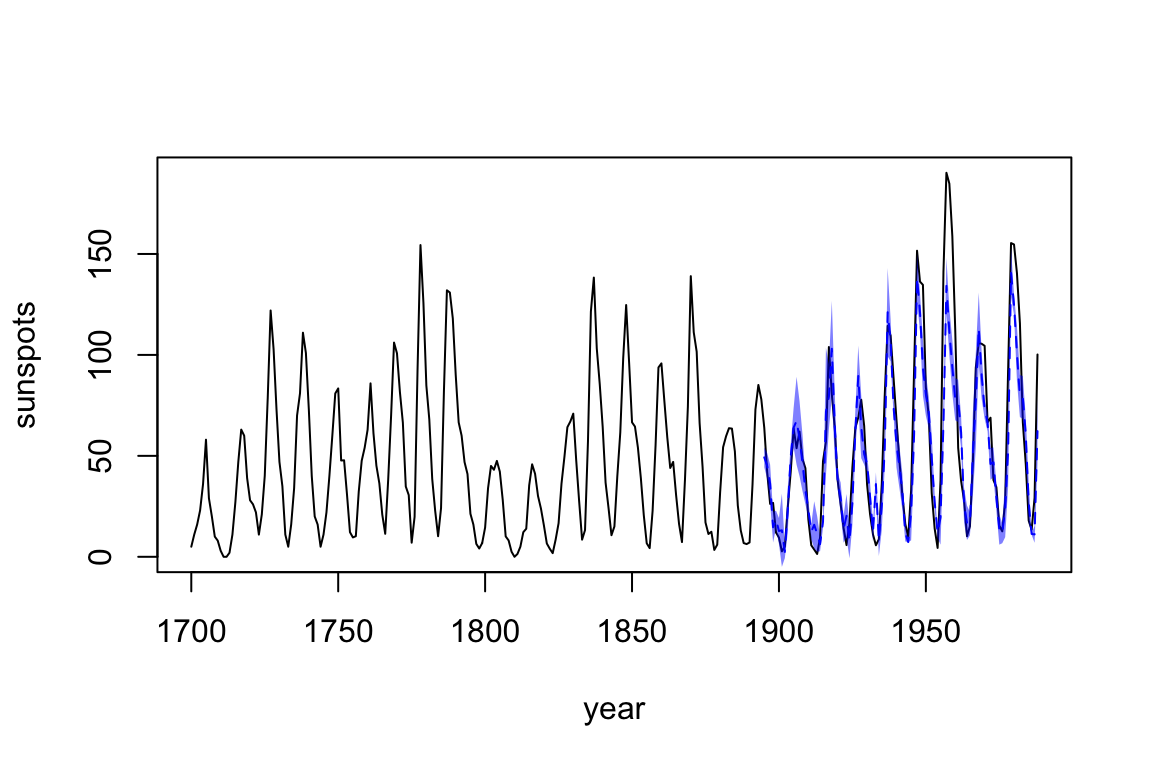Binder DemoOverview

The rEDM package is a collection of methods for Empirical Dynamic Modeling (EDM). EDM is based on the mathematical theory of recontructing attractor manifolds from time series data, with applications to forecasting, causal inference, and more. It is based on research software previously developed for the Sugihara Lab (University of California San Diego, Scripps Institution of Oceanography).

Installation

You can install rEDM from CRAN with:

OR from github with:

If you are on Windows, you may need to install Rtools first, so that you have access to a C++ compiler.

Example

We begin by looking at annual time series of sunspots:

dat <- data.frame(yr = as.numeric(time(sunspot.year)),
sunspot_count = as.numeric(sunspot.year))

plot(dat$yr, dat$sunspot_count, type = "l",
xlab = "year", ylab = "sunspots")First, we use simplex to determine the optimal embedding dimension, E:

It looks like E = 3 or 4 is optimal. Since we generally want a simpler model, if possible, let’s go with E = 3 to forecast the remaining 1/3 of the data.

output <- simplex(dat,
lib = lib, pred = pred, # predict on last 1/3
E = 3,
stats_only = FALSE)     # return predictions, too

predictions <- na.omit(output$model_output[]) plot(dat$yr, dat$sunspot_count, type = "l", xlab = "year", ylab = "sunspots") lines(predictions$time, predictions$pred, col = "blue", lty = 2) polygon(c(predictions$time, rev(predictions$time)), c(predictions$pred - sqrt(predictions$pred_var), rev(predictions$pred + sqrt(predictions\$pred_var))),
col = rgb(0, 0, 1, 0.5), border = NA)Further Examples

Please see the package vignettes for more details: